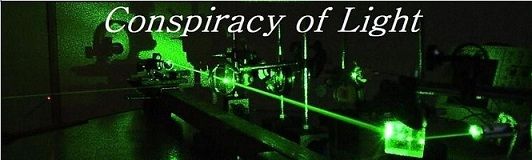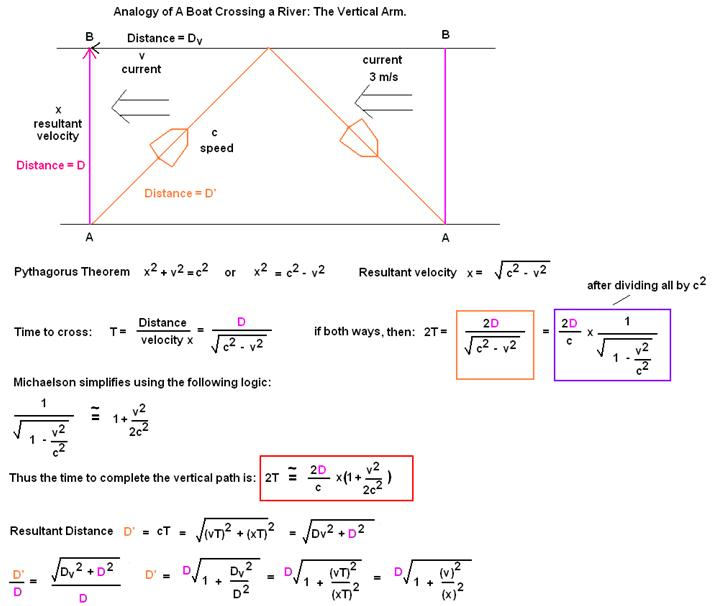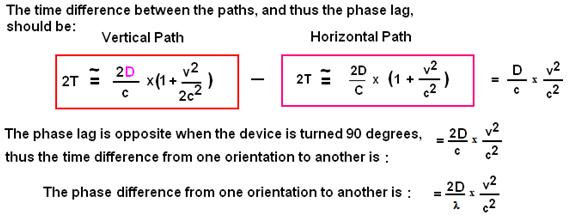The search for  new physics.

The Michelson-Morley Experiment (1887)

Doug Marett (2010)

In the later part of the 19th century, the undulatory theory of light assumed the existence of a medium called the ether, whose vibrations produce the phenomenon of heat and light, and which is supposed to fill all space. According to Fresnel, the ether, which is enclosed in optical media, partakes of the motion of these media, to an extent depending on their indices of refraction. Assuming then that the ether is at rest, the Earth moving through it, the time required for light to pass from one point to another on the Earth’s surface, will depend on the direction in which it travels.” So was the premise of Albert Michelson in his 1881 paper which sought to test for the existence of ether drift – the flow of ether past the Earth as the Earth travels through space. Michelson and Morley devised a method by which to test this hypothesis. This consisted of the well known Michelson Interferometer as shown below in Fig. 1A.

Fig. 1A:This instrument sought to detect the motion of the Earth through a static ether, as the Earth moved through its orbit around the sun at 30km/s. Since the relative movement of the ether past the Earth would be in the opposite direction of the Earth’s velocity, this might be conceived of as an ether wind, that would tend to carry the light in that direction past the instrument. By creating two different light paths, one parallel to this flow (horizontal arm) , and the other perpendicular to this flow (vertical arm), the ether drift past the instrument would slow the light by two different amounts, which would result in the two light waves re-uniting back at the detector out-of phase, in an amount proportional to the velocity difference between the Earth and the ether. This method can be explained using the analogy of a boat crossing a stream vs. a boat running back and forth through a stream.

Fig. 1BThe equations show that both the horizontal path and the vertical path should both be longer, proportional to the velocity of the Earth in space, and thus the time that light takes to complete each path is also longer. However, the horizontal path was expected to be longer in time than the vertical path by (D/c)(v2/c2) . When the interferometer was shifted 90 degrees, this fringe shift is reversed. Thus the total fringe shift was anticipated to be on the order of (2D/l)(v2/c2). As is well known, this experiment showed no difference between the phase of the light traveling in the vertical arm vs. the horizontal arm, when turned 90 degrees with respect to the motion of the Earth through space.

When the Michelson - Morley Experiment is considered in isolation, at least three theories are consistent with a null result:

1) Lorentz Ether Theory (LET)

The Lorentz ether theory proposes a single preferred frame for light (a static ether). In modern terms, this frame would correspond to the frame of the cosmic microwave background (CMB) radiation. Fitzgerald, Larmor, and finally Lorentz proposed that the null result was due to a length contraction of the horizontal arm in the direction of motion, by an amount proportional to (1/2D)(v2/c2) = (1-v2/c2)1/2, this becoming known as the Lorentz contraction. In this proposal, the horizontal path is shortened forward and return (total D * v2/c2) and the opposite way for the 90 degree turn of the apparatus, for a complete shortening of 2D * v2/c2, which exactly nullifies any effect of an ether wind. This contraction hypothesis becomes the first tenant of any ether theory based on a "conspiracy of light" - namely a theory that must include that the properties of light and matter are such that matter must contract in exactly the right amount to obscure any evidence of a medium of space such as a preferred reference frame for light.

2) Relativity Theory

Special Relativity Theory calls for light to isotropic in all inertial reference frames and for real time to be variable. For relativity, the Lorentz contraction is retained as a concept but is not a real contraction in the frame of the observer. It only appears to be a contraction to an observer moving relative to the first observer's frame, and is believed to be the result of real time-de-synchronization. This could aptly be called a "conspiracy of time" theory.

3) Gravitational Frame Dragging Theory:

Alternatively, the null result of the Michelson-Morley experiment can be explained based on an ether theory that assumes that the ether is somehow dragged or modified in its properties by gravitational fields. This type of theory is a theory of gravitational preferred frames. It relies on a different "conspiracy of light", this time gravity modifies the properties of light such that its velocity appears to be isotropic with respect to the moving gravitational frame. This type of theory may or may not involve a physical Lorentz contraction, since in such a model the anisotropy of light in the moving preferred frame is very small (on Earth, it would be due only at most to the rotation of the Earth - no more than 465 m/s). Since most optical experiments to date are only capable of measuring second order effects (where the measurable effect of anisotropy is on the order of v2/c2) a latitude dependent rotation velocity of say 365 m/s, which is 1000x less than the velocity of Earth with respect to the CMB, would result in an optical effect of 1 million times smaller in say an interferometer experiment. Further, this effect would go effectively to zero if the Lorentz contraction is real, unless the optical design is clever enough to be unaffected in principle.